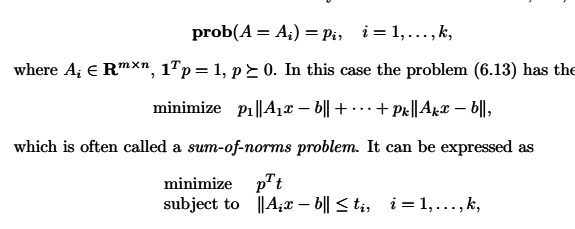# A sum-of-norms problem

Hi all！
I have a problem just like the sum-of-norms problem described in chapter 6.4: Robust approximation from the book Convex Optimation.
,Just like the above but use the Chebyshev norm and write it in Matlab as follows:

p=0.2.ones(5,1);
cvx_begin quiet
variable t(5) nonnegative
variable x(n)
minimize ( p’t )
subject to
norm(A1
x(n)-b, Inf)<=t(1,1);
norm(A2
x(n)-b, Inf)<=t(2,1);
norm(A3x(n)-b, Inf)<=t(3,1);
norm(A4
x(n)-b, Inf)<=t(4,1);
norm(A5*x(n)-b, Inf)<=t(5,1);
cvx_end

A1, A2, A3, A4, A5 are m plus n matrixes.

Run these codes and I get the error:
Error using cvx/plus (line 45)
Matrix dimensions must agree.

Error in cvx/minus (line 21)
z = plus( x, y, true, cheat );

Error in test_CVX(line 266)
norm(A1*x(n)-b, Inf)<=t(1,1);

Why？Any help would be appreciated! Thanks！

You want norm(A1*x-b, Inf)<=t(1,1);
x(n) is just the nth element of x, so you end up with an m by n matrix minus an m by 1 vector b, which is of incompatible dimensions, and of course not what you want.
Change the other norm lines as well.

BTW, you could just write t(1),t(2), etc., but what you have is o.k.

1 Like

Oh，sorry for my mistake，Thanks vey much and it works! Nice weekend！